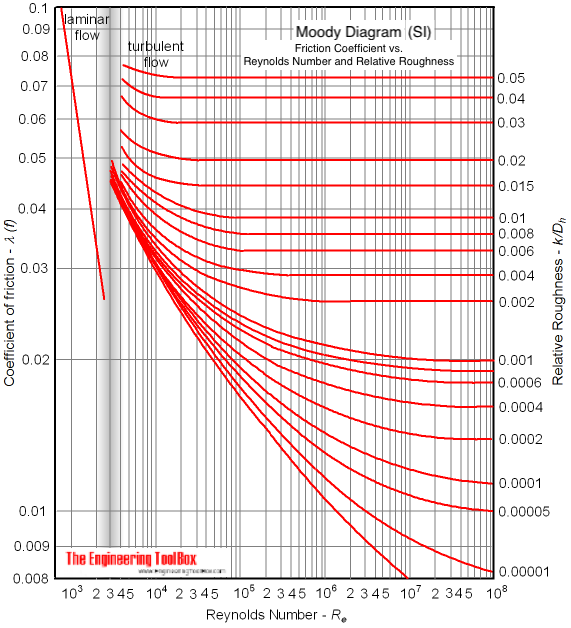Engineering ToolBox - Resources, Tools and Basic Information for Engineering and Design of Technical Applications!

# Laminar Flow - Friction Coefficients

## Calculate friction coefficients for laminar fluid flow.

The friction coefficient - or factor - of a fluid flow at laminar conditions can be calculated as

λ = 64 / Re

= 64 μ / (dh u ρ)

= 64 ν / (dh u)                          (1)

where

λ = friction coefficient (non-dimensional)

Re = Reynolds Number (non-dimensional)

μ = absolute (dynamic) viscosity  (Ns/m2, lbm/s ft)

dhhydraulic diameter (mm, ft)

u = mean velocity in flow (m/s, ft/s)

ρ = density of fluid (kg/m3, lbm/ft3  )

ν μ / ρkinematic viscosity (m2/s, ft2/s)

Equation (1) is only valid at laminar conditions where Reynolds Number is less than 2300. For turbulent conditions where Reynolds Number exceeds 4000 the Colebrook equation should be used to calculate the friction coefficient.

In practice laminar flow is only actual for viscous fluids - like crude oil, fuel oil and other oils.

The friction coefficient for laminar flow indicated in the Moody diagram (SI based):## Related Topics

• ### Fluid Mechanics

The study of fluids - liquids and gases. Involving velocity, pressure, density and temperature as functions of space and time.

## Related Documents

• ### Colebrook Equation

Friction loss coefficients in pipes, tubes and ducts.
• ### Darcy-Weisbach Equation - Major Pressure and Head Loss due to Friction

The Darcy-Weisbach equation can be used to calculate the major pressure and head loss due to friction in ducts, pipes or tubes.
• ### Reynolds Number

Introduction and definition of the dimensionless Reynolds Number - online calculators.

## Engineering ToolBox - SketchUp Extension - Online 3D modeling!

Add standard and customized parametric components - like flange beams, lumbers, piping, stairs and more - to your Sketchup model with the Engineering ToolBox - SketchUp Extension - enabled for use with older versions of the amazing SketchUp Make and the newer "up to date" SketchUp Pro . Add the Engineering ToolBox extension to your SketchUp Make/Pro from the Extension Warehouse !

We don't collect information from our users. More about

## Citation

• The Engineering ToolBox (2008). Laminar Flow - Friction Coefficients. [online] Available at: https://www.engineeringtoolbox.com/laminar-friction-coefficient-d_1032.html [Accessed Day Month Year].

Modify the access date according your visit.

9.29.12